## 4.2.3 Thermal Conductivity

The thermal conductivity is modeled by a power law: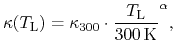where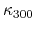is the value at 300 K. From early experiments=130 W/mK for bulk'' GaN  was extracted. However, later measurements of epitaxial structures yielded higher values , and a strong dependence on the dislocation density was observed . Based on various studies [292,293,295,296,297,298] we give two parameter sets in Table 4.4, applicable for different material quality. Fig. 4.1 compares the two model sets with other models and experimental data.

 Material[W/mK]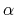GaN model 1 130 -0.43 GaN model 2 220 -1.2 AlN 350 -1.7 InN 45-176 0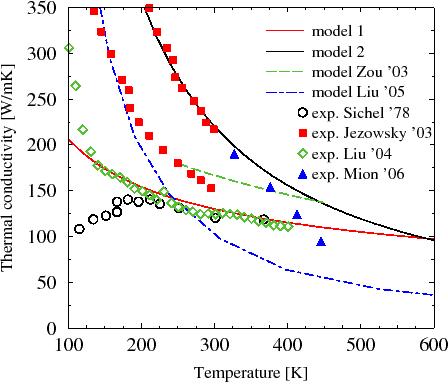For AlN the variation of the measured values for the thermal conductivity is smaller (Fig. 4.2). We assume=350 W/mK, which is close to the value reported in . The parameter, which models the decrease with temperature, is calibrated against measured data [299,300,301].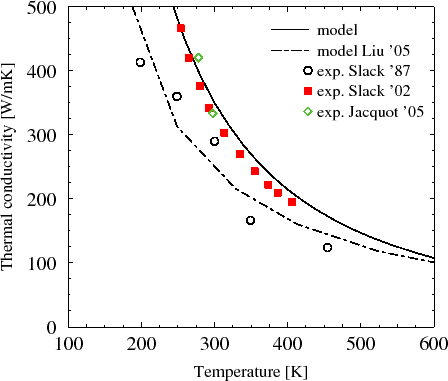As of today no studies of the temperature dependent thermal conductivity of InN are available. Based on  a=176 W/mK at 300 K is assumed. This is a theoretical estimation, while the measured value was only 45 W/mK due to phonon scattering by point-defects and grain-boundaries.

Several expressions exist for the thermal conductivity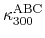of semiconductor alloys. As an example, Adachi et al.  use one based on Abeles's complex model . However, an even more straightforward approach is proposed in , where a harmonic mean is used to model the conductivity at 300 K, while the exponent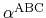is linearly interpolated as there is no experimental data for temperatures other than 300 K yet: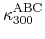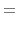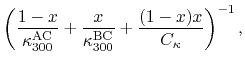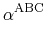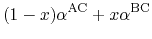Applying this expressions, a value of 3.1 W/mK is adopted for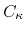of Al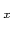Ga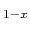N. This results in a fair agreement with the experimental data of Daly et al.  and Liu et al.  as depicted in Fig. 4.3 and corresponds to the value used in .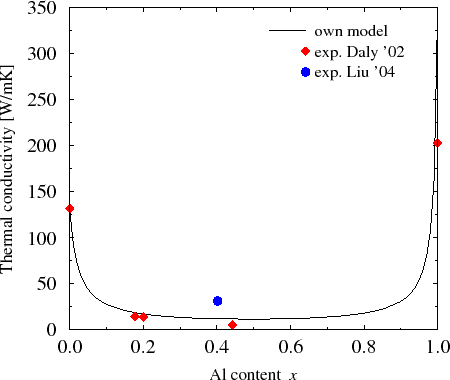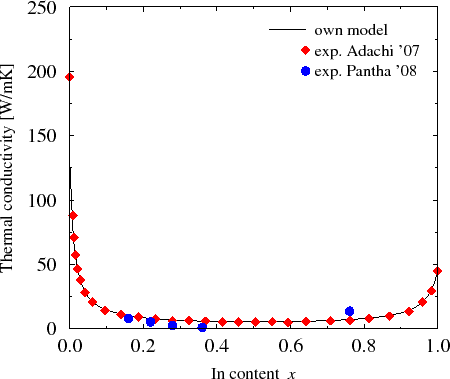For InGaN=1.5 W/mK is adopted, again matching the model in  (Fig. 4.4) and the experimental data of Pantha et al. . For InAlN a fit to the only available experimental data  resulted in an=1.2 W/mK (Fig. 4.5).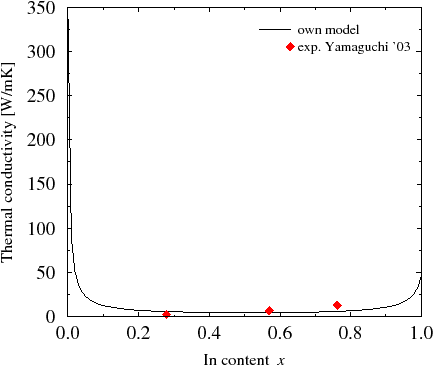S. Vitanov: Simulation of High Electron Mobility Transistors